# Expected goals from bookmaker odds

I recently read an interesting paper called The Betting Odds Rating System: Using soccer forecasts to forecast soccer by Wunderlich and Memmert. In their paper they develop av variant of the good old Elo rating system. Instead of using the actual outcomes of each match to calculate the ratings, they use the probabilities of the outcomes, which they get from bookmaker odds.

I was wondering if a similar approach could be used together with the goalmodel package I released a couple of months ago. The models available in the package are models I have written about extensively on this blog, and they all work as follows: You use the number of goals scored to get some ratings of the goal scoring and goal conceding rates of each team. You then use these ratings to forecast the expected number of goals in the upcoming games. These expected goals can then be used to calculate the probabilities of the outcome (Home win, draw, away win). A crucial step in these calculations is the assumption that the number of goals scored follow the Poisson distribution (or some related distribution, like the Negative Binomial).

But can we turn this process the other way around, and use bookmaker odds (or odds from other sources) to get expected goals and maybe also attack and defense ratings like we do in the goalmodel package? I think this is possible. I have written a function in R that takes outcome probabilities and searches for a pair of expected goals that matches the probabilities. You can find it on github. This function relies on using the Poisson distribution.

Next, I have expanded the functionality of the goalmodel package so that you can use expected goals for model fitting instead of just observed goals. This is possible by setting the model argument to “model = ‘gaussian'” or to “model = ‘ls'”. These two options are currently experimental, and are a bit unstable, so if you use them, make sure to check if the resulting parameter estimates make sense.

I used my implied package to convert bookmaker odds from the 2015-16 English Premier League into probabilities (using the power method), found the expected goals, and then fitted a goalmodel using the least squares method. Here are the resulting parameters, from both using the expected goals and observed goals: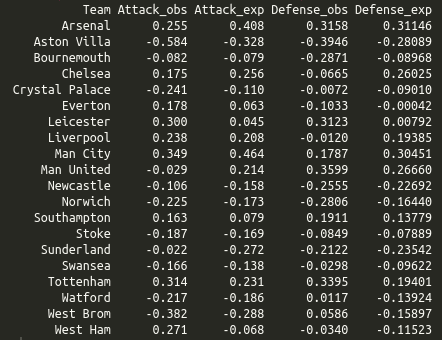I wanted to use this season for comaprison as this was the season Leicester won unexpectedly, and in the Odds-Elo paper (figure 6) it seemed like the ratings based on the odds were more stable than the ones based on the actual results, which increased drastically during the season. In the attack and defense ratings from the goalmodels we see that Leicester have average ratings (which is what ratings close to 0 are) in the model based on odds, and much higher ratings based on the actual results. So the goalmodel and Elo ratings seem to agree, basically.

I also recently discovered another paper titled Combining historical data and bookmakers’odds in modelling football scores, that tries something similar as I have done here. They seem to do the same extraction of the expected goals from the bookmaker odds as I do, but they don’t provide the details. Instead of using the expected goals to fit a model, they fit a model based on actual scores (similar to what the goalmodel package do), and then they take a weighted average of the model based expected goals and the expected goals from the bookmaker odds.

# Introducing the goalmodel R package

I have written a lot about different models for forecasting football results, and provided a lot of R code along the way. Especially popular are my posts about the Dixon-Coles model, where people still post comments, four years since I first wrote them. Because of the interest in them, and the interest in some of the other models I have written about, I decided to tidy up my code and functions a bit, and make an R package out of it. The result is the goalmodel R package. The package let you fit the ordinary Poisson model, which was one of the first models I wrote about, the Dixon-Coles model, The Negative-Binomial model, and you can also use the adjustment I wrote about in my previous update.

The package contains a function to fit the different models, and you can even combine different aspects of the different models into the same model. You can for instance use the Dixon-Coles adjustment together with a negative binomial model. There is also a range of different methods for making prediction of different kinds, such as expected goals and over/under.

The package can be downloaded from github. It is still just the initial version, so there are probably some bugs and stuff to be sorted out, but go and try it out and let me know what you think!

# A simple re-implementation of the Dixon-Coles model

A couple of years ago I implemented the Dixon-Coles model for predicting football results here on this blog. That series of of blog posts is my most popular since I keep getting comments on it, some four years later.

One of the most common requests is advice on how to expand the model to include additional predictors. Unfortunately with the implementation I posted this was not so straightforward. It relied on some design matrices with dummy-coded variables, which is a standard way of doing things in regression modeling. The DC model isn’t a standard regression modeling problem, so using matrices complicated things. I posted some updates and variant across several posts, which in the end made the code a bit hard to follow and to modify.

Anyway, I’ve had a simpler implementation lying around for a while, and since there’s been far between updates on this blog lately I thought I’d post it.

First load some data from the engsoccerdata package. I’m going to use the 2011-12 season of the English Premier League, so the results can be compared with what I got from the first implementation.

library(dplyr)
library(engsoccerdata)

england %>%
filter(Season == 2011,
tier==1) %>%
mutate(home = as.character(home),
visitor = as.character(visitor))-> england_2011


Next we should create a list of initial parameter values. This will be used as a starting point for estimating the parameters. The list contains vectors of four groups of parameters, the attack and defense parameters of all teams, the home field advantage and the Dixon-Coles adjustment (rho). The attack and defense vector are named so that it is easy to look up the relevant parameter later on.

Notice also that a sum-to-zero constraint has to be added to the defense parameters, so in reality we are estimating one defense parameter less than the number of teams. Check this post for some more explanation of this.

# Make a vector of all team names.
all_teams <- sort(unique(c(england_2011$home, england_2011$visitor)), decreasing = FALSE)
n_teams <- length(all_teams)

# list of parameters with initial values.
parameter_list <- list(attack = rep(0.2, n_teams),
defense = rep(-0.01, n_teams-1),
home = 0.1,
rho= 0.00)

names(parameter_list$attack) <- all_teams names(parameter_list$defense) <- all_teams[-1] # the first parameter is computed from the rest.


Next we need a function that calculates the negative log-likelihood function, to be used with R’s built in optimizer.

One trick I use here is to relist the parameters. The optimizer want all parameter values as a single vector. When you have a lot of parameters that group together and is used in different parts of the model, this can quickly create some complicated indexing and stuff. By supplying the original parameter list, plus having named vectors, these problems essentially disappear.

Also notice how the expected goals are now simply computed by looking up the relevant parameters in the parameter list and adding them together. No need for matrix multiplications.

The Dixon-Coles adjustment function tau is the same as in the original implementation.

dc_negloglik <- function(params, goals_home, goals_visitor,
team_home, team_visitor, param_skeleton){

# relist, to make things easier.
plist <- relist(params, param_skeleton)

# There is a sum-to-zero constraint on defense parameters.
# The defense parameter for the first team is computed from the rest.
plist$defense <- c(sum(plist$defense)*-1, plist$defense) names(plist$defense) <- names(plist$attack) # add name to first element. # Home team expected goals lambda_home <- exp(plist$attack[team_home] + plist$defense[team_visitor] + plist$home)

# Away team expected goals
lambda_visitor <- exp(plist$attack[team_visitor] + plist$defense[team_home])

dc_adj <- tau(goals_home, goals_visitor, lambda_home, lambda_visitor, rho = plist$rho) # Trick to avoid warnings. if (any(dc_adj <= 0)){ return(Inf) } # The log-likelihood log_lik_home <- dpois(goals_home, lambda = lambda_home, log=TRUE) log_lik_visitor <- dpois(goals_visitor, lambda = lambda_visitor, log=TRUE) log_lik <- sum((log_lik_home + log_lik_visitor + log(dc_adj))) return(log_lik*-1) }  To actually estimate the parameters we feed the function, data and initial values to optim, and check the results.  optim_res <- optim(par = unlist(parameter_list), fn=dc_negloglik, goals_home = england_2011$hgoal,
goals_visitor = england_2011$vgoal, team_home = england_2011$home, team_visitor = england_2011$visitor, param_skeleton=parameter_list, method = 'BFGS') # relist, and calculate the remaining parameter. parameter_est <- relist(optim_res$par, parameter_list)
parameter_est$defense <- c( sum(parameter_est$defense) * -1, parameter_est$defense) names(parameter_est$defense) <- names(parameter_est\$attack)


I get the same home field advantage (0.27) and rho (-0.13) as in the original implementation. The other parameters differ, however. This is because of the sum-to-zero constraints are coded in a different way. This should not matter and both ways should give the same predictions.

I have not yet said anything about how to expand the model to include other predictors, but hopefully this implementation should make it easier. You can just add some new arguments to the dc_negloglik function that takes the variables in question as input, and add new parameter vectors to the parameter list as needed. Then the calculations of the expected goals should be modified to include the new parameters and predictors.

# Fitting Bradley-Terry models using Stan

I have recently played around with Stan, which is an excellent software to fit Bayesian models. It is similar to JAGS, which I have used before to fit some regression models for predicting football results. Stan differs from JAGS in a number of ways. Although there is some resemblance between the two, the model specification languages are not compatible with each other. Stan, for instance, uses static typing. On the algorithmic side, JAGS uses the Gibbs sampling technique to sample from the posterior; Stan does not do Gibbs sampling, but has two other sampling algorithms. In Stan you can also get point estimates by using built-in optimization routines that search for the maximum of the posterior distribution.

In this post I will implement the popular Bradley-Terry machine learning model in Stan and test it on some sports data (handball, to be specific).

The Bradley-Terry model is used for making predictions based on paired comparisons. A paired comparison in this context means that two things are compared, and one of them is deemed preferable or better than the other. This can for example occur when studying consumer preferences or ranking sport teams.

The Bradley-Terry model is really simple. Suppose two teams are playing against each other, then the probability that team i beats team j is

$$p(i > j) = \frac{r_i}{r_i + r_j}$$

where $$r_i$$ and $$r_j$$ are the ratings for the two teams, and should be positive numbers. It is these ratings we want to estimate.

A problem with the model above is that the ratings are not uniquely determined. To overcome this problem the parameters need to be constrained. The most common constraint is to add a sum-to-one constraint

$$\sum_k r_k = 1$$

I will explore a different constraint below.

Sine we are in a Bayesian setting we need to set a prior distribution for the rating parameters. Given the constraints that the parameters should be positive and sum-to-one the Dirichlet distribution is a natural choice of prior distribution.

$$r_1, r_2, …, r_p \sim Dir(\alpha_1, \alpha_2, …, \alpha_p)$$

where the hyperparameters $$\alpha$$ are positive real numbers. I will explore different choices of these below.

Here is the Stan code for the Bradley-Terry model:

data {
int<lower=0> N; // N games
int<lower=0> P; // P teams

// Each team is referred to by an integer that acts as an index for the ratings vector.
int team1[N]; // Indicator arrays for team 1
int team2[N]; // Indicator arrays for team 1
int results[N]; // Results. 1 if team 1 won, 0 if team 2 won.

vector[P] alpha; // Parameters for Dirichlet prior.
}

parameters {
// Vector of ratings for each team.
// The simplex constrains the ratings to sum to 1
simplex[P] ratings;
}

model {
real p1_win[N]; // Win probabilities for player 1

ratings ~ dirichlet(alpha); // Dirichlet prior.

for (i in 1:N){
p1_win[i] = ratings[team1[i]] / (ratings[team1[i]] + ratings[team2[i]]);
results[i] ~ bernoulli(p1_win[i]);
}
}


The way I implemented the model you need to supply the hyperparameters for the Dirichlet prior via R (or whatever interface you use to run Stan). The match outcomes should be coded as 1 if team 1 won, 0 if team 2 won. The two variables team1 and team2 are vectors of integers that are used to reference the corresponding parameters in the ratings parameter vector.

Before we fit the model to some data we need to consider what values we should give to the hyperparameters. Each of the parameters of the Dirichlet distribution corresponds to the rating of a specific team. Both the absolute magnitude and the relative magnitudes are important to consider. A simple case is when all hyperparameters have the same value. Setting all hyperparameters to be equal to each other, with a value greater or equal to 1, implies a prior belief that all the ratings are the same. If they are between 0 and 1, the prior belief is that the ratings are really really different. The magnitude also plays a role here. The greater the magnitudes are, the stronger the prior belief that the ratings are the same.

Let’s fit some data to the model. Below are the results from fitting the results from 104 games from the 2016-17 season of the Norwegian women’s handball league, with 11 participating teams. I had to exclude six games that ended in a tie, since that kind of result is not supported by the Bradley-Terry model. Extension exists that handle this, but that will be for another time.

Below are the results of fitting the model with different sets of priors, together with the league points for comparison. For this purpose I didn’t do any MCMC sampling, I only found the MAP estimates using the optimization procedures in Stan.For all the priors the resulting ratings give the same ranking. This ranking also corresponds well with the ranking given by the league points, except for Gjerpen and Stabæk which have switched place. We also clearly see the effect of the magnitude of the hyperparameters. When all the $$\alpha$$‘s are 1 the ratings varies from almost 0 to about 0.6. When they are all set to 100 the ratings are almost all the same. If these ratings were used to predict the result of future matches the magnitudes of the hyperparameters could be tuned using cross validation to find the value that give best predictions.

What if we used a different hyperparameter for each team? Below are the results when I set all $$\alpha$$‘s to 10, except for the one corresponding to the rating for Glassverket, which I set to 25. We clearly see the impact. Glassverket is now considered to be the best team. This is nice since it demonstrates how actual prior information, if available, can be used to estimate the ratings.I also want to mention another way to fit the Bradley-Terry model, but without the sum-to-one constraint. The way to do this is by using a technique that the Stan manual calls soft centering. Instead of having a Dirichlet prior which enforces the constraint, we use a normal distribution prior. This prior will not give strict bounds on the parameter values, but will essentially provide a range of probable values they can take. For the model I chose a prior with mean 20 and standard deviation 6.

$$r_1, r_2, …, r_p \sim N(\mu = 20, \sigma = 6)$$

The mean hyperprior here is arbitrary, but the standard deviation required some more considerations. I reasoned that the best team would probably be in the top 99 percentile of the distribution, approximately three standard deviations above the mean. In this case this would imply a rating of 20 + 3*6 = 38. Similarly, the worst team would probably be rated three standard deviations below the mean, giving a rating of 2. This implies that the best team has a 95% chance of winning against the worst team.

Here is the Stan code:

data {
int<lower=0> N;
int<lower=0> P;

int team1[N];
int team2[N];
int results[N];

real<lower=0> prior_mean; // sets the (arbitrary) location of the ratings.
real<lower=0> prior_sd; // sets the (arbitrary) scale of the ratings.

}

parameters {
real<lower=0> ratings[P];
}

model {
real p1_win[N];

// soft centering (see stan manual 8.7)
ratings ~ normal(prior_mean, prior_sd);

for (i in 1:N){
p1_win[i] = ratings[team1[i]] / (ratings[team1[i]] + ratings[team2[i]]);
results[i] ~ bernoulli(p1_win[i]);
}
}


And here are the ratings for the handball teams. The ratings are now on a different scale than before and largely matches the prior distribution. The ranking given by this model agrees with the model with the Dirichlet prior, with Gjerpen and Stabek switched relative to the league ranking.# Which model is the best?

I had a discussion on Twitter a couple of weeks ago about which model is the best for predicting football results. I have suspected that the Dixon & Coles model (DC), which is a modification of the Poisson model, tend to overfit. Hence it should not generalize well and give poorer predictions. I have written about one other alternative to the Poisson model, namely the Conway-Maxwell Poisson model (COMP). This is a model for count data that can be both over-, equi- and underdispersed. It is basically a Poisson model but without the assumption that the variance equals the mean. I have previously done some simple analyses comparing the Poisson, DC and COMP models, and concluded then that the COMP model was superior. The analysis was however a bit to simple, so I have now done a more proper evaluation of the models.

A proper way to evaluatie the models is to do a backtest. For each day there is a game played, the three models are fitted to the available historical data (but not data from the future, that would be cheating) and then used to predict the match outcomes for that day. I did this for two leagues, the English Premier League and German Bundesliga. The models were fitted to data from both the top league and the second tier divisions, since this improves the models, but only the results of the top division was predicted and used in the evaluation. I used a separate home field advantage for the two divisions and the rho parameter in the DC model and the dispersion parameter in the COMP model was estimated using the top division only.

To measure the model’s predictive ability I used the Ranked Probability Score (RPS). This is the proper measure to evaluate predictions for the match outcome in the form of probabilities for home win, draw and away win. The range of the RPS goes from 0 (best possible predictions) to 1 (worst possible prediction). Since the three models actually model the number of goals, I also looked at the probability they gave for the actual score.

For all three models I used the Dixon & Coles method to weight the historical data that is used in training the models. This requires tuning. For both the English and German leagues I backtested the models on different values of the weighting parameter $$\xi$$ on the seasons from 2005-06 to 2009-10, with historical data available from 1995. I then used the optimal $$\xi$$ for backtesting the seasons 2010-11 up to December 2016. This last validation period covers 1980 Bundesliga matches and 2426 Premier League matches.

Here are the RPS for the three models plottet against $$\xi$$. Lower RPS is better and lower $$\xi$$ weights more recent data higher.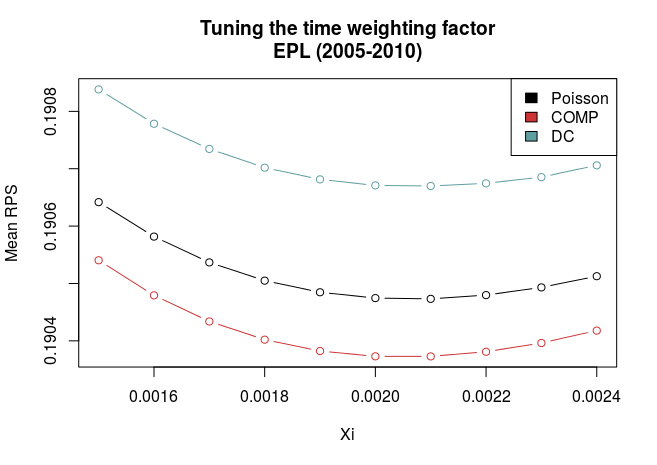The graphs show a couple of things. First, all three models have best predictive ability at the same value of $$\xi$$, and that they compare similarly also for non-optimal values of $$\xi$$. This makes things a bit easier since we don’t have to worry that a different value of $$\xi$$ will alter our evaluations about which model is the best.

Second, there is quite some difference between the models for the German and English data. In the English data the COMP model is clearly best, while the DC is the worst. In the German league, the DC is clearly better, and the COMP and Poisson models are pretty much equally good.

So I used the optimal values of $$\xi$$ (0.0021 and 0.0015 for Premier League and Bundesliga, respectively) to validate the models in the data from 2010 and onwards.

Here is a table of the mean RPS for the three models: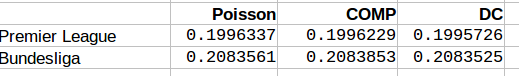We see that for the both English Premier League and German Bundesliga the DC model offers best predictions. The COMP model comes second in Premier League, but has worst performance in the Bundesliga. It is interesting that the DC model performed worst in the tuning period for the Premier League, now was the best one. For the Bundesliga the models compared similarly as in the tuning period.

I also looked at how often the DC and COMP models had lower RPS than the Poisson model. The results are in this table: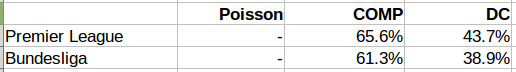The COMP model outperformed the Poisson model in more than 60% of the matches in both leagues, while the DC model did so only about 40% of the time.

When looking at the goal scoring probabilities. Here is a table of the sum of the minus log probabilities for the actual scoreline. Here a lower number also indicates better predictions.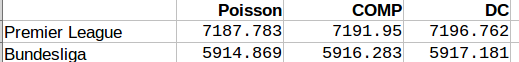Inn both the Premier League and Bundesliga the Poisson model was best, followed by COMP, with the DC model last.

We can also take a look at the parameter values for the extra parameters the DC and COMP models has. Remember that the DC models is becomes the Poisson model when rho = 0, while the COMP model is the same as the Poisson model when upsilon = 1, and is underdispersed when upsilon is greater than 1.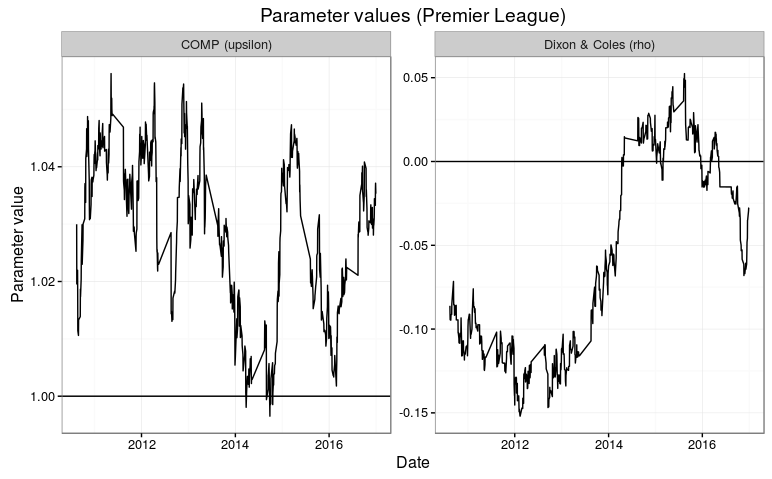The parameter estimates fluctuates a bit. It is intersting to see that the rho parameter in the DC model tend to be below 1, which gives the opposite direction of what Dixon and Coles found in their 1997 paper. In the Premier League, the parmater makes a big jump to above 0 at the end of the 2013-14 season. The parameter appears to be a bit more consistent in the Bundesliga, but also there we see a short period where the parameter is around 0.

The dispseriosn parameter upsilon also isn’t all that consistent. It is generally closer to 1 in the Bundesliga than in the Premier League. I think this is consistent with why this model was better in the Premier League than in the Bundesliga.

All inn all I think it is hard to conclude which of the three models is the best. The COMP and DC models both adjusts the Poisson model in their own specific ways, and this may explain why the different ways of measuring their predictive abilities are so inconsistent. The DC model seem to be better in the German Bundesliga than in the English Premier League. I don’t think any of the two models are generally better than the ordinary Poisson model, but it could be worthwhile to look more into when the two models are better, and perhaps they could be combined?

# Calculate the ranked probability score in R

I was asked in the comments for the R code for the ranked probability score, so instead of posting it deep down in the comments I thought I’d post it as a proper blog instead. The ranked probability score (RPS) is a measure of how similar two probability distributions are and is used as a way to evaluate the quality of a probabilistic prediction. It is an example of a proper scoring rule.

The RPS was brought to my attention in the paper Solving the problem of inadequate scoring rules for assessing probabilistic football forecasting models by Constantinou and Fenton. In that paper they argue that the RPS is the best measure of the quality of football predictions when the predictions are of the type where you have probabilities for the outcome (home win, draw or away win). The thing about the RPS is that it also reflects that an away win is in a sense closer to a draw than a home win. That means that a higher probability predicted for a draw is considered better than a higher probability for home win if the actual result is an away win.

You can also find some more details at the pena.lt/y blog.

The following R function takes two arguments. The first argument (predictions) is a matrix with the predictions. It should be laid out so that each row is one prediction, laid out in the proper order, where each element is a probability and each row sum to 1. The second argument (observed) is a numeric vector that indicates which outcome that was actually observed.

For assessing football predictions the predictions matrix would have three columns, with the probabilities for the match ordered as home, draw and away (or in the opposite order).

rankProbScore <- function(predictions, observed){
ncat <- ncol(predictions)
npred <- nrow(predictions)

rps <- numeric(npred)

for (rr in 1:npred){
obsvec <- rep(0, ncat)
obsvec[observed[rr]] <- 1
cumulative <- 0
for (i in 1:ncat){
cumulative <- cumulative + (sum(predictions[rr,1:i]) - sum(obsvec[1:i]))^2
}
rps[rr] <- (1/(ncat-1))*cumulative
}
return(rps)
}


# Underdispersed Poisson alternatives seem to be better at predicting football results

In the previous post I discussed some Poisson-like probability distributions that offer more flexibility than the Poisson distribution. They typically have an extra parameter that controls the variance, or dispersion. The reason I looked into these distributions was of course to see if they could be useful for modeling and predicting football results. I hoped in particular that the distributions that can be underdispersed would be most useful. If the underdispersed distributions describe the data well then the model should predict the outcome of a match better than the ordinary Poisson model.

The model I use is basically the same as the independent Poisson regression model, except that the part with the Poisson distribution is replaced by one of the alternative distributions. Let the $$Y_{ij}$$ be the number of goals scored in game i by team j

$$Y_{ij} \sim f(\mu_{ij}, \sigma)$$
$$log(\mu_{ij}) = \gamma + \alpha_j + \beta_k$$

where $$\alpha_j$$ is the attack parameter for team j, and $$\beta_k$$ is the defense parameter for opposing team k, and $$\gamma$$ is the home field advantage parameter that is applied only if team j plays at home. $$f(\mu_{ij}, \sigma)$$ is one of the probability distributions discussed in the last post, parameterized by the location parameter mu and dispersion parameter sigma.

To these models I fitted data from English Premier League from the 2010-11 season to the 2014-15 season. I also used Bundesliga data from the same seasons. The models were fitted separately for each season and compared to each other with AIC. I consider this only a preliminary analysis and I have therefore not done a full scale testing of the accuracy of predictions where I refit the model before each match day and use Dixon-Coles weighting.

The five probability distributions I used in the above model was the Poisson (PO), negative binomial (NBI), double Poisson (DPO), Conway-Maxwell Poisson (COM) and the Delaporte (DEL) which I did not mention in the last post. All of these, except the Conway-Maxwell Poisson, were easy to fit using the gamlss R package. I also tried two other gamlss-supported models, the Poisson inverse Gaussian and Waring distributions, but the fitting algorithm did not work properly. To fit the Conway-Maxwell Poisson model I used the CompGLM package. For good measure I also fitted the data to the Dixon-Coles bivariate Poisson model (DC). This model is a bit different from the rest of the models, but since I have written about it before and never really tested it I thought this was a nice opportunity to do just that.

The AIC calculated from each model fitted to the data is listed in the following table. A lower AIC indicates that the model is better. I have indicated the best model for each data set in red.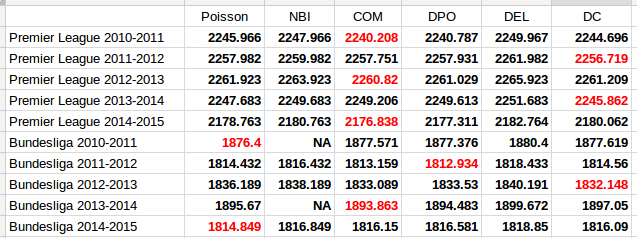The first thing to notice is that the two models that only account for overdispersion, the Negative Binomial and Delaporte, are never better than the ordinary Poisson model. The other and more interesting thing to note, is that the Conway-Maxwell and Double Poisson models are almost always better than the ordinary Poisson model. The Dixon-Coles model is also the best model for three of the data sets.

It is of course necessary to take a look at the estimates of the parameters that extends the three models from the Poisson model, the $$\sigma$$ parameter for the Conway-Maxwell and double Poisson and the $$\rho$$ for the Dixon-Coles model. Remember that for the Conway-Maxwell a $$\sigma$$ greater than 1 indicates underdispersion, while for the Double Poisson model a $$\sigma$$ less than 1 is indicates underdispersion. For the Dixon-Coles model a $$\rho$$ less than 0 indicates an excess of 0-0 and 1-1 scores and fewer 0-1 and 1-0 scores, while it is the opposite for $$\rho$$ greater than 0.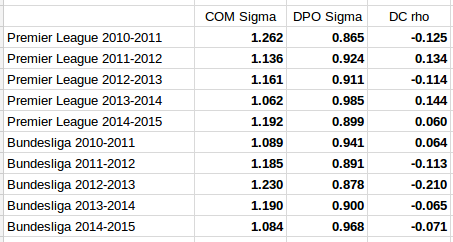It is interesting to see that the estimated dispersion parameters indicate underdispersion for all the data sets. It is also interesting to see that the data sets where the parameter estimates are most indicative of equidispersion is where the Poisson model is best according to AIC (Premier League 2013-14 and Bundesliga 2010-11 and 2014-15).

The parameter estimates for the Dixon-Coles model do not give a very consistent picture. The sign seem to change a lot from season to season for the Premier League data, and for the data sets where the Dixon-Coles model was found to be best, the signs were in the opposite direction of what where the motivation described in the original 1997 paper. Although it does not look so bad for the Bundesliga data, this makes me suspect that the Dixon-Coles model is prone to overfitting. Compared to the Conway-Maxwell and double Poisson models that can capture more general patterns in all of the data, the Dixon-Coles model extends the Poisson model to just parts of the data, the low scoring outcomes.

It would be interesting to do fuller tests of the prediction accuracy of these three models compared to the ordinary Poisson model.

# Some alternatives to the Poisson distribution

One important characteristic of the Poisson distribution is that both its expectation and the variance equals parameter $$\lambda$$. A consequence of this is that when we use the Poisson distribution, for example in a Poisson regression, we have to assume that the variance equals the expected value.

The equality assumption may of course not hold in practice and there are two ways in which this assumption can be wrong. Either the variance is less than the expectation or it is greater than the expectation. This is called under- and overdispersion, respectively. When the equality assumption holds, it is called equidispersion.

There are two main consequences if the assumption does not hold: The first is that standard errors of the parameter estimates, which are based on the Poisson, are wrong. This could lead to wrong conclusions when doing inference. The other consequence happens when you use the Poisson to make predictions, for example how many goals a football team will score. The probabilities assigned to each number of goals to be scored will be inaccurate.

(Under- and overdispersion should not be confused with heteroscedasticity in ordinary linear regression. Poisson regression models are naturally heteroscedastic because of the variance-expectation equality. Dispersion refers to what relationship there is between the variance and the expected value, in other words what form the heteroscedasticity takes.)

When it comes to modeling and predicting football results using the Poisson, a good thing would be if the data were actually underdispersed. That would mean that the probabilities for the predicted number of goals scored would be higher around the expectation, and it would be possible to make more precise predictions. The increase in precision would be greatest for the best teams. Even if the data were really overdispersed, we would still get probabilities that more accurately reflect the observed number of goals, although the predictions would be less precise.

This is the reason why I have looked into alternatives to the Poisson model that are suitable to model count data and that are capable of being over- and underdispersed. Except for the negative binomial model there seems to have been little focus on more flexible Poisson-like models in the literature, although there are a handful of papers from the last 15 years with some applied examples.

I should already mention the gamlss package, which is an extremely useful package that can fit a large number of regression type models in R. I like to think of it as the glm function on steroids. It can be used to create regression models for a large number of distributions (50+) and using different forms of dependent variables (for example random effects and splines) and doing regression on distribution parameters other than the usual expectation parameters.

The models that I have considered usually have two parameters. The two parameters are often not easy to interpret, but the distributions can be re-parameterized (which is done in the gamlss package) so that the parameters describe the location (denoted $$\mu$$, often the same as the expectation) and shape (denoted $$\sigma$$, often a dispersion parameter that modifies the association between the expectation and variance). Another typical property is that they equal the Poisson for certain values of the shape parameter.

As I have already mentioned, the kind of model that is most often put forward as an alternative to the Poisson is the Negative binomial distribution (NBI). The advantages of the negative binomial are that is well studied and good software packages exists for using it. The shape parameter $$\sigma > 0$$ determines the overdispersion (relative to the Poisson) so that the closer it is to 0, the more it resembles the Poisson. This is a disadvantage as it can not be used to model underdispersion (or equidispersion, although in practice it can come arbitrarily close to it). Another similar, but less studied, model is the Poisson-inverse Gaussian (PIG). It too has a parameter $$\sigma > 0$$ that determines the overdispersion.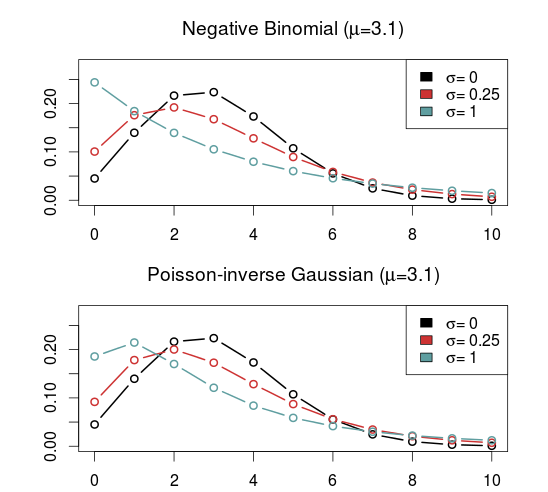A large class of distributions, called Weighted Poisson distributions, is capable of being both over- and underdispersed. (The terms Weighted in the name comes from a technique used to derive the distribution formulas, not that the data is weighted) A paper describing this class can be found here. The general form of the probability distribution is

$$P(x;\theta,\alpha)=\frac{e^{\mu x+\theta t(x)}}{x!C(\theta,\alpha)}$$

where $$t(x)$$ is one of a large number of possible functions, and $$C(\theta,\alpha)$$ is a normalizing constant which makes sure all probabilities in the distribution sum to 1. Note that I have denoted the two parameters using $$\theta$$ and $$\alpha$$ and not $$\mu$$ and $$\sigma$$ to indicate that these are not necessarily location and shape parameters. I think this and interesting class of distributions that I want to look more into, but since they are not generally implemented in any R package that I know of I will not consider them further now.

Another model that is capable of being over- and underdispersed is the Conway–Maxwell–Poisson distribution (COM), which incidentally is a special case of the class of Weighted Poisson distributions mentioned above (see this paper). The Poisson distribution is a special case of the COM when $$\sigma = 1$$, and is underdispersed when $$\sigma > 1$$ and overdispersed when $$\sigma$$ is between 0 and 1. One drawback with the COM model is that the expected value depends on both parameters $$\mu$$ and $$\sigma$$, although it is dominated by $$\mu$$. This makes the interpretation a bit difficult, but it may not be a problem when making predictions.

Unfortunately, the COM model is not supported by the gamlss package, but there are some other R packages that implements it. I have tried a few of them and the only one that I got to work is CompGLM, which for some reason does not use the location ($$\mu$$) and shape ($$\sigma$$) parameterization.The Double Poisson (DP) is another interesting distribution which also equals the Poisson distribution when $$\sigma = 1$$, but is overdispersed when $$\sigma > 1$$ and underdispersed when $$\sigma$$ is between 0 and 1. The expectation does not depend on the shape parameter $$\sigma$$, and it is approximately equal to the location parameter $$\mu$$. Another interesting thing about the Double Poisson is that it is belongs to a larger group of distributions called double exponential families which also lets you derive a binomial-like distribution with an extra dispersion parameter which can be useful in a logistic regression setting (see this paper, or this preprint).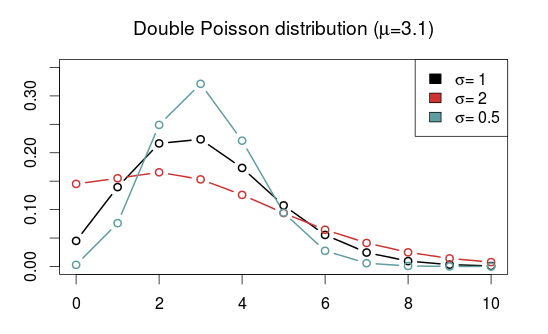In a follow up post I will try to use these distributions in regression models similar to the independent Poisson model.

# Some thoughts on goal differences in football matches without draws

In regular league matches, draws are a common occurrence. Modeling and predicting draws have some complications. Elo-type ratings allows for draws by simply treating them as half-wins for each team, but it does not allow for direct calculation of draw probabilities. Poisson regression models naturally lets you figure out the probability of a draw by calculating the probability of a goal difference of zero.

Poisson models have the additional strength over Elo-type systems in that they can be used to model and predict the number of goals scored, not only who wins (or loose, or draws). The models I have looked at all assumes that draws are possible, and that is the case in regular league matches. But what about the matches where draws are not allowed, such as in knockout tournaments? How could you calculate probabilities for different number of goals?

I haven’t really seen any discussion about this anywhere, but I have this one idea I just want to get out there. Bear in mind that this idea I present here is completely untested, so I can not say for sure if it is any good.

Matches where draws are impossible are a minority of the matches, so building and fitting a separate model for just those matches is not a good idea. Instead I propose an adjustment to be applied for just those matches. The adjustment can be motivated as follows: The game starts with 0-0 between the teams, so at least one goal has to be scored. This should increase the probabilities of 0-1 and 1-0 results. Similar argument can be given to a game that is in a 1-1 state, a 2-2 state, and so on; at least one goal has to be scored.

So the adjustment is to simply divide the probabilities for a draw and add them to the probabilities for a one-goal difference. This should of course be illustrated with an example.

Suppose you have a matrix with goal probabilities. This can be computed using a Poisson regression model, perhaps with the Dixon-Coles adjustment or some other bivariate structure, or perhaps it comes from a completely different kind of model. It doesn’t really matter.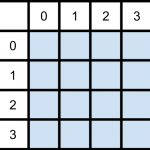Then it is just to divide the draw probabilities and add them to the appropriate cells in the matrix: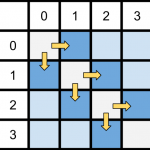But how should the probabilities be divided? We could just divide them evenly between the two teams, but I think it is more appropriate to divide them based on the relative strengths of the two teams. There are may ways this could be done, but I think a reasonable method is to divide them based on the win-probabilities for the two teams (given that there is no draw). This does not rely on anything other than the goal probability matrix itself, and is easy to compute: Take the sum of the upper and lower triangle of the matrix, divided by the sum of the whole matrix except the diagonal. This also maintains the original win/lose probabilities.

This scheme is easy to implement in R. First we need a matrix of probabilities, which I here just compute using two Poisson distributions, then calculate the win probability of the team with goals on the vertical. After that we divide the diagonal with the win-probabilities.

# Matrix of goal probabilities
probability_matrix <- dpois(0:7, 1.1) %*% t(dpois(0:7, 1.6))

# Win probabilities, for dividing the draw probabilities
prop <- sum(mm[lower.tri(mm)]) / (1 - sum(diag(mm)))

# Diagonal values, split proportionally
divided_vertical <- (diag(probability_matrix) * prop)
divided_horizontal <- (diag(probability_matrix) * (1-prop))


Here we encounter a problem. The two vectors we are going to add to the two secondary diagonals are one element too long. If we have a big enough probability matrix, that last element is probably going to be so small that ignoring it should not matter too much.

# Increase the probabilities for one-goal wins.
diag(probability_matrix[-1,]) <- diag(probability_matrix[-1,]) + divided_vertical[-length(divided_vertical)]
diag(probability_matrix[,-1]) <- diag(probability_matrix[,-1]) + divided_horizontal[-length(divided_horizontal)]

# The main diagonal, with draw probabilities, should be 0.
diag(mm) <- 0


As always, it is nice to see how the probabilities of the goal differences are distributed. Here I have plotted the adjusted and unadjusted probability distributions: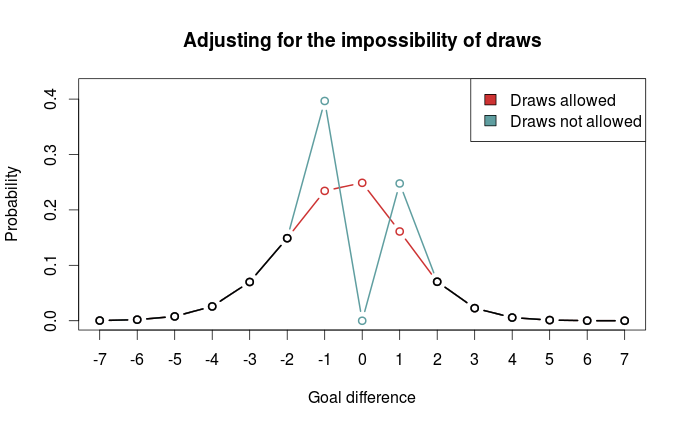We clearly see that one-goal wins are much more probable.

As I mentioned above, I haven’t really looked at any data, and it is quite possible that other adjustments are better. Perhaps boosting one-goal wins is a poor idea, and spreading the probabilities more out would be better.

# The Dixon-Coles approach to time-weighted Poisson regression

In the previous blog posts about predicting football results using Poisson regression I have mostly ignored the fact that the data points (ie matches) used to fit the models are gathered (played) at different time points.

In the 1997 Dixon and Coles paper where they described the bivariate adjustment for low scores they also discussed using weighted maximum likelihood to better make the parameter estimates reflect the current abilities of the teams, rather than as an average over the whole period you have data from.

Dixon and Coles propose to weight the games using a function so that games are down weighted exponentially by how long time it is since they were played. The function to determine the weight for a match played is

$$\phi(t) = exp(-\xi t)$$

where t is the time since the match was played, and $$\xi$$ is a positive parameter that determines how much down-weighting should occur.

I have implemented this function in R, but I have done a slight modification from the one from the paper. Dixon and Coles uses “half weeks” as their time unit, but they do not describe in more detail what exactly they mean. They probably used Wednesday or Thursday as the day a new half-week starts, but I won’t bother implementing something like that. Instead I am just going to use days as the unit of time.

This function takes a vector of the match dates (data type Date) and computes the weights according to the current date and a value of $$\xi$$. The currentDate argument lets you set the date to count from, with all dates after this will be given weight 0.

DCweights <- function(dates, currentDate=Sys.Date(), xi=0){
datediffs <- dates - as.Date(currentDate)
datediffs <- as.numeric(datediffs *-1)
w <- exp(-1*xi*datediffs)
w[datediffs <= 0] <- 0 #Future dates should have zero weights
return(w)
}


We can use this function to plot how the much weight the games in the past is given for different values of $$\xi$$. Here we see that $$\xi = 0$$ gives the same weight to all the matches. I have also set the currentDate as a day in April 2013 to illustrate how future dates are weighted 0.To figure out the optimal value for $$\xi$$ Dixon and Coles emulated a situation where they predicted the match results using only the match data prior to the match in question, and then optimizing for prediction ability. They don’t explain how exactly they did the optimizing, but I am not going to use the optim function to do this. Instead I am going to just try a lot of different values of $$\xi$$, and go for the one that is best. The reason for this is that doing the prediction emulation takes some time, and using an optimizing algorithm will take an unpredictable amount of time.

I am not going to use the Dixon-Coles model here. Instead I am going for the independent Poisson model. Again, the reason is that I don’t want to use too much time on this.

To measure prediction ability, Dixon & Coles used the predictive log-likelihood (PLL). This is just the logarithm of the probabilities calculated by the model for the outcome that actually occurred, added together for all matches. This means that a greater PLL indicates that the actual outcomes was more probable according to the model, which is what we want.

I want to use an additional measure of prediction ability to complement the PLL: The ranked probability score (RPS). This is a measure of prediction error, and takes on values between 0 and 1, with 0 meaning perfect prediction. RPS measure takes into account the entire probability distribution for the three outcomes, not just the probability of the observed outcome. That means a high probability of a draw is considered less of an error that a high probability of away win, if the actual outcome was home win. This measure was popularized in football analytics by Anthony Constantinou in his paper Solving the Problem of Inadequate Scoring Rules for Assessing Probabilistic Football Forecast Models. You can find a link to a draft version of that paper on Constantinou’s website.

I used data from the 2005-06 season and onwards, and did predictions from January 2007 and up until the end of 2014. I also skipped the ten first match days at the beginning of each season to avoid problems with lack of data for the promoted teams. I did this for the top leagues in England, Germany, Netherlands and France. Here are optimal values of $$\xi$$ according to the two prediction measurements:

PLL RPS
England 0.0018 0.0018
Germany 0.0023 0.0023
Netherlands 0.0019 0.0020
France 0.0019 0.0020

The RPS and PLL mostly agree, and where they disagree, it is only by one in the last decimal place.

Dixon & Coles found an optimal value of 0.0065 in their data (which were from the 1990s and included data from the top four English leagues and the FA cup), but they used half weeks instead of days as their time unit. Incidentally, if we divide their value by the number of days in a half week (3.5 days) we get 0.00186, about the same I got. The German league has the greatest optimum value, meaning historical data is of less importance when making predictions.

An interesting thing to do is to plot the predictive ability against different values of $$\xi$$. Here are the PLL (adjusted to be between 0 and 1, with the optimum at 1) for England and Germany compared, with their optima indicated by the dashed vertical lines:I am not sure I want to interpret this plot too much, but it does seems like predictions for the German league are more robust to values of $$\xi$$ greater than the optimum, as indicated by the slower decline in the graph, than the English league.

So here I have presented the values of $$\xi$$ for the independent Poisson regression model, but will these values be the optimal for the Dixon & Coles model? Probably not, but I suspect there will be less variability between the two models than between the same model fitted for different leagues.